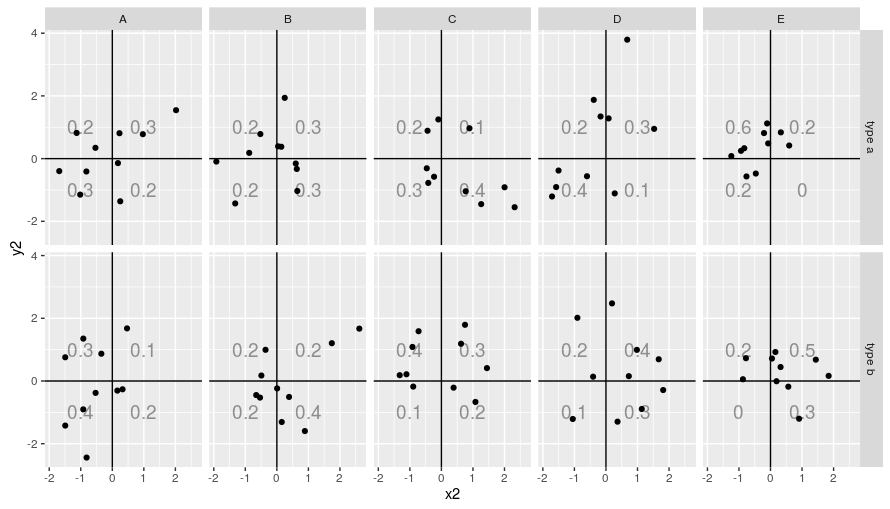# R Code Snippets

## R Code Snippets

### Grouping and counting objects

#### Using `table`

```df <- data.frame(name = c("a", "a", "a", "b", "b", "c", "c", "c", "c"), x = 1:9)
tt <- table(df\$name)
tt
```
```
a b c
3 2 4
```

#### Using `dplyr`

```library(dplyr)
df <- data.frame(name = c("a", "a", "a", "b", "b", "c", "c", "c", "c"), x = 1:9)
tt <- df %>% group_by(name) %>% summarize( count = n() )
tt
```
```# A tibble: 3 x 2
name  count
<fct> <int>
1 a         3
2 b         2
3 c         4
```

### `ggplot2`

#### Adding labels in a multi-faceted graph

I originally wrote this code to answer a question on Stackoverflow. Another user was quicker than me (and provided a more beautiful answer) but I want to save my version.

```df <- data.frame(
x2=rnorm(100),
y2=rnorm(100),
type = c(rep("type a", 50),
rep("type b", 50)),
kind = rep(LETTERS[1:5],10))

library(ggplot2)
library(plyr)

group_size = nrow(dat)
lq1 <- nrow( subset( dat, x2 >= 0 & y2 >= 0))
lq2 <- nrow( subset( dat, x2 >= 0 & y2  < 0))
lq3 <- nrow( subset( dat, x2  < 0 & y2  < 0))
lq4 <- nrow( subset( dat, x2  < 0 & y2 >= 0))
quadrant4 = lq4 / group_size )
}

labels <- ddply(df, c("type", "kind"), quadrant_labels)

ggplot(df, aes(x2, y2)) + geom_point() +
geom_vline(xintercept = 0) +
geom_hline(yintercept = 0) +
geom_text(data = labels, x =  1, y =  1, aes(label = quadrant1), alpha=.40, size=5) +
geom_text(data = labels, x =  1, y = -1, aes(label = quadrant2), alpha=.40, size=5) +
geom_text(data = labels, x = -1, y = -1, aes(label = quadrant3), alpha=.40, size=5) +
geom_text(data = labels, x = -1, y =  1, aes(label = quadrant4), alpha=.40, size=5) +
facet_grid(type~kind)
```### Other sources of information

#### Cleaning the table

A small case study for tidyverse functions (`separate`, `pivot_longer` and `pivot_wider`, among the others)

Date: 2017-02-16 Thu 00:00

Created: 2020-01-17 Fri 15:11

Validate